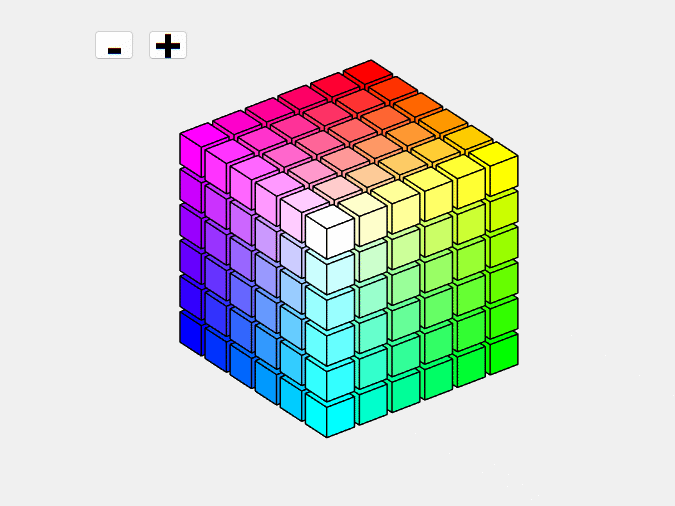This is a crosspost from   Cleve’s Corner: Cleve Moler on Mathematics and Computing Cleve Moler is the author of the first MATLAB, one of the founders of MathWorks, and is currently Chief Mathematician at the company. He writes here about MATLAB, scientific computing and interesting mathematics.. See the original post here.

# An Interactive Version of colorcubes

A 231-character static version of colorcubes in the current MiniHack generated quite a bit of interest. So I am posting the full version, where you can change number of cubes and the view point, at this link.

### Contents

#### Animation#### Quiz

What is the color of the cubelet, or cubelets, in the center?

Get the MATLAB code

Published with MATLAB® R2022a